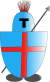# Sistance's EXPLODE!AI - depth-first search

Diese Seite auf Deutsch

My first attempt to develop a computer based enemy for Sistance's EXPLODE! led me to depth-first search with a minimax algorithm. The procedure is very simple and the weighting function can be simple, too.

As I wrote at the AI development main site, a forecasting AI must test a minimum number of moves to discover a special combination as dangerous. In Sistance's EXPLODE! a field can have a value between 0 and 5 and can be possessed by one of two players. (A field with a value of 0 is possessed by no one. But this value cannot be reached again once it is possessed by a player.)
In every turn a player will increase the value of one field by 1. So it takes up to 4 turns to increase the value of a field from 1 to 5 - and one more turn to let that field explode (and attack fields next to it). Because the two players act alternately, the AI has to calculate 9 "half moves" before it can decide (or recognize) if it is an advantage to increase the value of that field.

Basically spoken, the depth-first search algorithm tries to calculate every single movement combination until it reaches a predefined search depth and then tries to weight the given state. In doing so it calculates a complete movement order with a predefined number of half moves before it calculates the next movement order which it hasn't already been weighted.
The algorithm must cache only a few number of movement combinations. The minimax algorithm, that is used to determine the best move in depth-first search, allows to dump all former movement combinations, as soon as it recovered a better (or a worse) move.

Technically the algorithm is checking every possible movement combination unoptimized. The needed calculation time may increase exponentially with the number of half moves. This is a big disadvantage. The testing system needed several seconds for just 5 half moves. The needed time for the target number of 10 or more half moves would have been unacceptable long.

Because of that I gave the breadth-first search a chance - an algorithm with some interesting optimization possibilities.